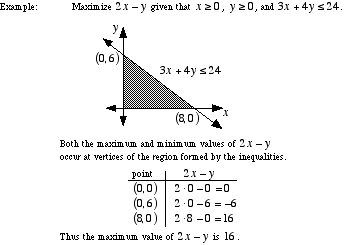index: click on a letter A B C D E F G H I J K L M N O P Q R S T U V W X Y Z A to Z index index: subject areas numbers & symbols sets, logic, proofs geometry algebra trigonometry advanced algebra & pre-calculus calculus advanced topics probability & statistics real world applications multimedia entrieswww.mathwords.com about mathwords website feedback

 Linear Programming An algorithm for solving problems asking the largest or smallest possible value of a linear polynomial. Any restrictions on the problem must be expressed as a system of inequalities; in particular, all equations and/or inequalities must be linear. Note: The region defined by the system will always by convex.